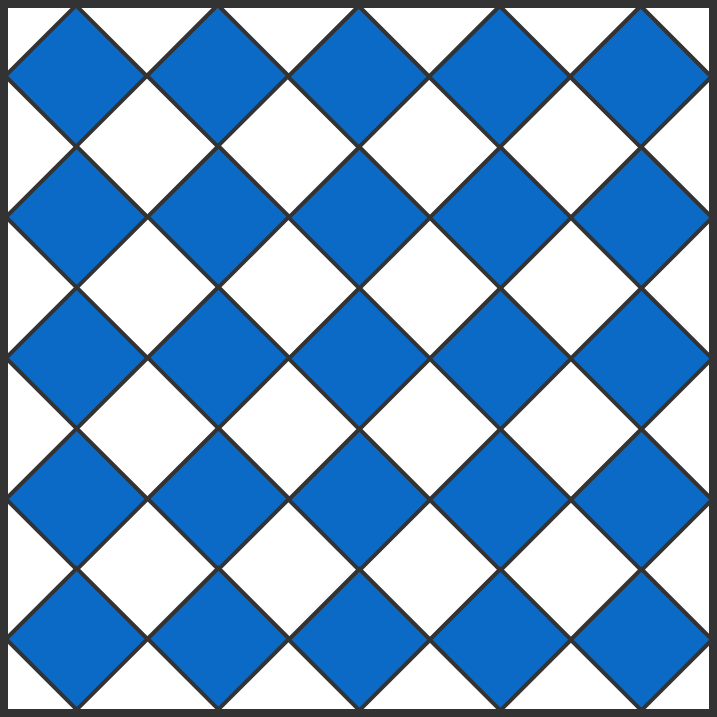# Problems of the Week

Contribute a problem

# 2017-09-11 BasicThe area of the large square is 100 and all of the small, blue squares are congruent.

What is the total area shaded blue?

Which is larger, $\sqrt{ 5 + \frac5{24} }\quad \text{ or }\quad 5 \times \sqrt{\frac5{24} }\,?$A wrecking ball is a heavy metal mass that's swung, from a crane, into structures to demolish them. Suppose we release a ball of mass $M$ from initial angle $\theta$ and measure the speed at the lowest point in its swing to be $v_\textrm{max}.$

If we replace the wrecking ball with one of mass $2M,$ keeping the length of the chain and the angle $\theta$ at which the ball is released constant, how will $v_\textrm{max}$ be affected?


Details and Assumptions:

• Neglect air resistance.
• The mass of the chain is zero.

Two players are playing a shortened version of badminton: a 6-point, 3-game match with no deuce. Specifically, in each game, the player who first scores 6 points wins. The winner of the match is the player who first wins 2 out of 3 games.

Is it possible for the loser to have accumulated more points than the winner?

$\large 1 \; \square \; \dfrac{1}{2} \; \square \; \dfrac{1}{3}\; \square \; \dfrac{1}{4}\; \square \; \dfrac{1}{5} \; \square \; \dfrac{1}{6} \; \square \; \dfrac{1}{7} \; \square \; \dfrac{1}{8} \; \square \; \dfrac{1}{9} \; \square \; \dfrac{1}{10} \; \square \; \dfrac{1}{11} \; \square \; \dfrac{1}{12}= 0$

Can you fill the boxes with $+ \text{ and } -$ to make this equation true?

×